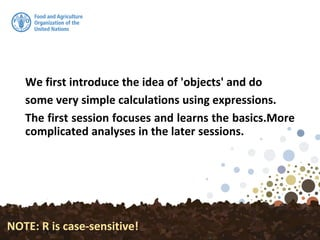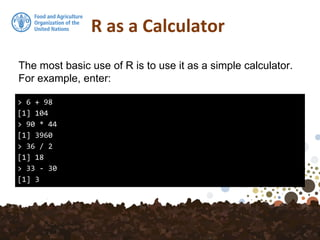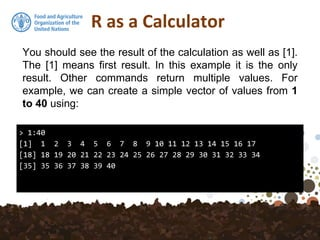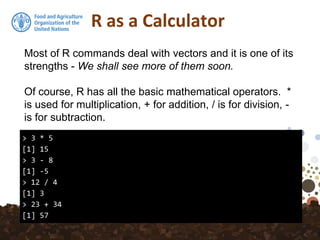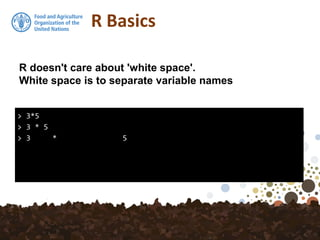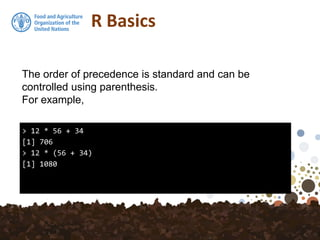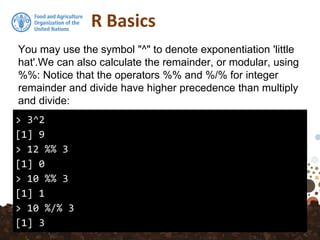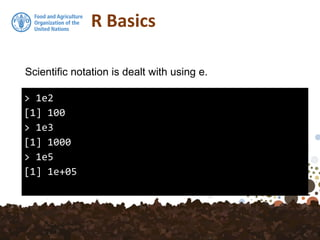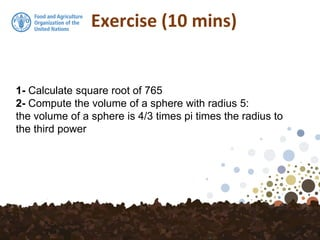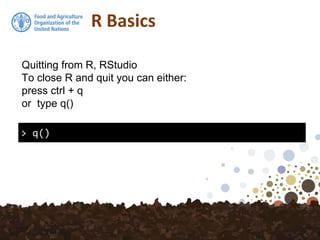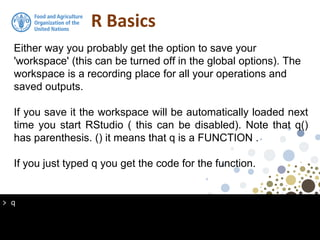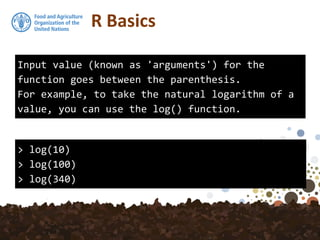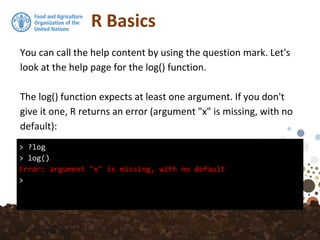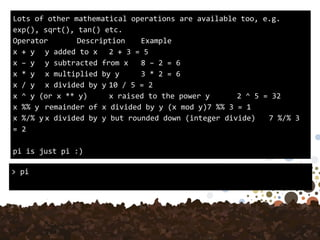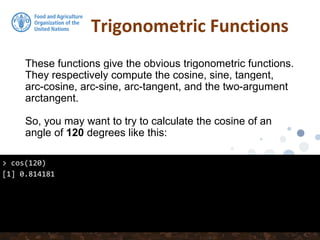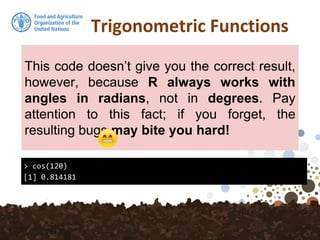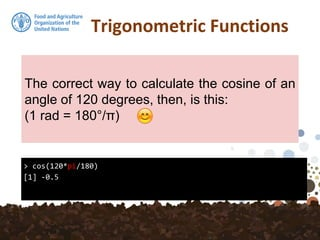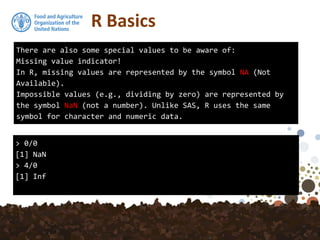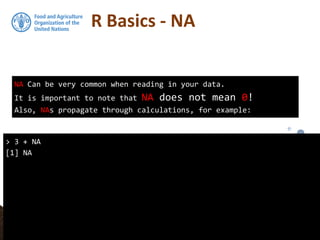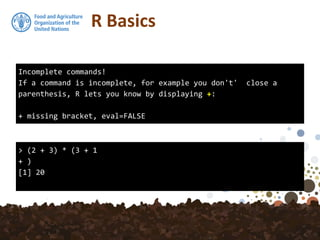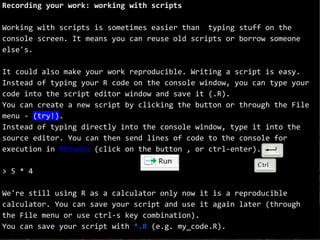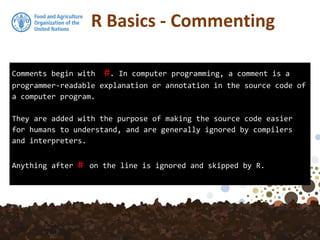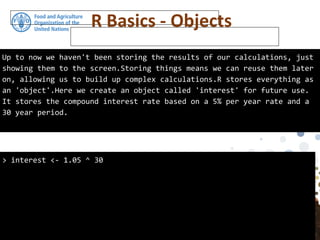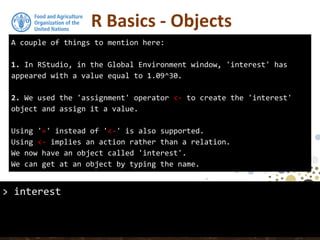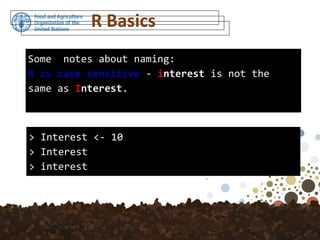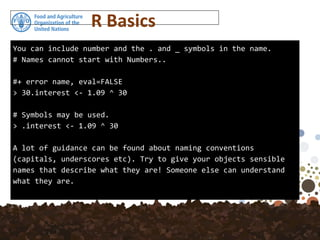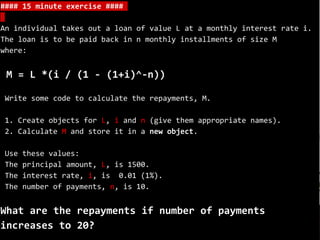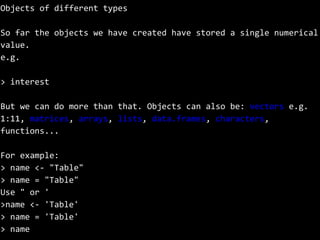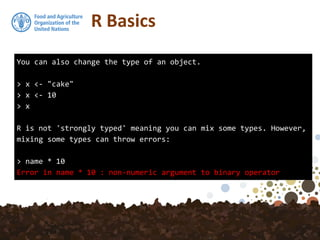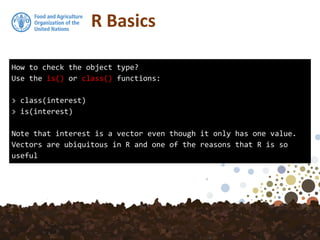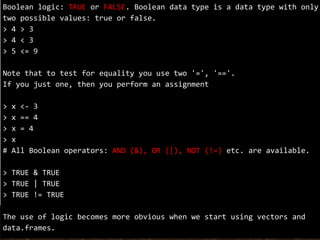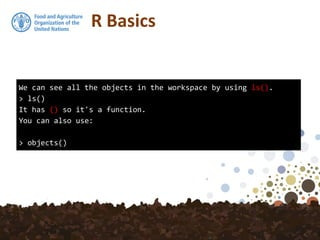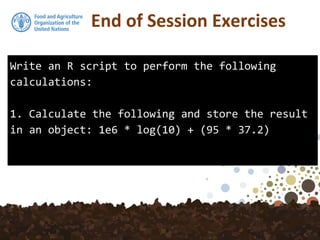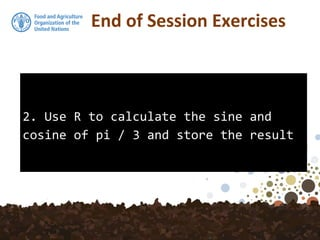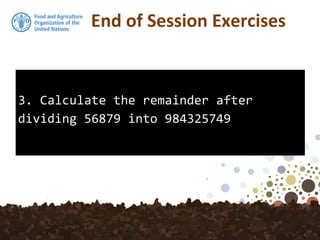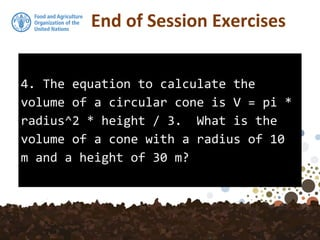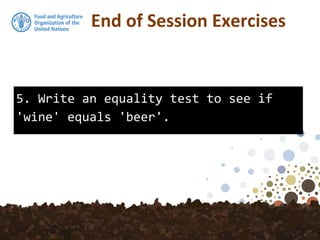1 de 40

### R basics

1. FAO- Global Soil Partnership Training on Digital Soil Organic Carbon Mapping 20-24 January 2018 Tehran/Iran Yusuf YIGINI, PhD - FAO, Land and Water Division (CBL) Guillermo Federico Olmedo, PhD - FAO, Land and Water Division (CBL)
2. Acquiring R Skills R Basics
3. We first introduce the idea of 'objects' and do some very simple calculations using expressions. The first session focuses and learns the basics.More complicated analyses in the later sessions. NOTE: R is case-sensitive!
4. R as a Calculator > 6 + 98  104 > 90 * 44  3960 > 36 / 2  18 > 33 - 30  3 The most basic use of R is to use it as a simple calculator. For example, enter:
5. R as a Calculator > 1:40  1 2 3 4 5 6 7 8 9 10 11 12 13 14 15 16 17  18 19 20 21 22 23 24 25 26 27 28 29 30 31 32 33 34  35 36 37 38 39 40 You should see the result of the calculation as well as . The  means first result. In this example it is the only result. Other commands return multiple values. For example, we can create a simple vector of values from 1 to 40 using:
6. R as a Calculator > 3 * 5  15 > 3 - 8  -5 > 12 / 4  3 > 23 + 34  57 Most of R commands deal with vectors and it is one of its strengths - We shall see more of them soon. Of course, R has all the basic mathematical operators. * is used for multiplication, + for addition, / is for division, - is for subtraction.
7. R Basics > 3*5 > 3 * 5 > 3 * 5 R doesn't care about 'white space'. White space is to separate variable names
8. R Basics > 12 * 56 + 34  706 > 12 * (56 + 34)  1080 The order of precedence is standard and can be controlled using parenthesis. For example,
9. R Basics > 3^2  9 > 12 %% 3  0 > 10 %% 3  1 > 10 %/% 3  3 You may use the symbol "^" to denote exponentiation 'little hat'.We can also calculate the remainder, or modular, using %%: Notice that the operators %% and %/% for integer remainder and divide have higher precedence than multiply and divide:
10. R Basics > 1e2  100 > 1e3  1000 > 1e5  1e+05 Scientific notation is dealt with using e.
11. Exercise (10 mins) 1- Calculate square root of 765 2- Compute the volume of a sphere with radius 5: the volume of a sphere is 4/3 times pi times the radius to the third power
12. R Basics > q() Quitting from R, RStudio To close R and quit you can either: press ctrl + q or type q()
13. > q R Basics Either way you probably get the option to save your 'workspace' (this can be turned off in the global options). The workspace is a recording place for all your operations and saved outputs. If you save it the workspace will be automatically loaded next time you start RStudio ( this can be disabled). Note that q() has parenthesis. () it means that q is a FUNCTION . If you just typed q you get the code for the function.
14. R Basics Input value (known as 'arguments') for the function goes between the parenthesis. For example, to take the natural logarithm of a value, you can use the log() function. > log(10) > log(100) > log(340)
15. R Basics > ?log > log() Error: argument "x" is missing, with no default > You can call the help content by using the question mark. Let's look at the help page for the log() function. The log() function expects at least one argument. If you don't give it one, R returns an error (argument "x" is missing, with no default):
16. R BasicsLots of other mathematical operations are available too, e.g. exp(), sqrt(), tan() etc. Operator Description Example x + y y added to x 2 + 3 = 5 x – y y subtracted from x 8 – 2 = 6 x * y x multiplied by y 3 * 2 = 6 x / y x divided by y 10 / 5 = 2 x ^ y (or x ** y) x raised to the power y 2 ^ 5 = 32 x %% y remainder of x divided by y (x mod y)7 %% 3 = 1 x %/% y x divided by y but rounded down (integer divide) 7 %/% 3 = 2 pi is just pi :) > pi
17. Trigonometric Functions These functions give the obvious trigonometric functions. They respectively compute the cosine, sine, tangent, arc-cosine, arc-sine, arc-tangent, and the two-argument arctangent. So, you may want to try to calculate the cosine of an angle of 120 degrees like this: > cos(120)  0.814181
18. Trigonometric Functions > cos(120)  0.814181 This code doesn’t give you the correct result, however, because R always works with angles in radians, not in degrees. Pay attention to this fact; if you forget, the resulting bugs may bite you hard!
19. Trigonometric Functions > cos(120*pi/180)  -0.5 The correct way to calculate the cosine of an angle of 120 degrees, then, is this: (1 rad = 180°/π)
20. R Basics There are also some special values to be aware of: Missing value indicator! In R, missing values are represented by the symbol NA (Not Available). Impossible values (e.g., dividing by zero) are represented by the symbol NaN (not a number). Unlike SAS, R uses the same symbol for character and numeric data. > 0/0  NaN > 4/0  Inf
21. R Basics - NA NA Can be very common when reading in your data. It is important to note that NA does not mean 0! Also, NAs propagate through calculations, for example: > 3 + NA  NA
22. R Basics Incomplete commands! If a command is incomplete, for example you don't' close a parenthesis, R lets you know by displaying +: + missing bracket, eval=FALSE > (2 + 3) * (3 + 1 + )  20
23. Recording your work: working with scripts Working with scripts is sometimes easier than typing stuff on the console screen. It means you can reuse old scripts or borrow someone else's. It could also make your work reproducible. Writing a script is easy. Instead of typing your R code on the console window, you can type your code into the script editor window and save it (.R). You can create a new script by clicking the button or through the File menu - (try!). Instead of typing directly into the console window, type it into the source editor. You can then send lines of code to the console for execution in RStudio (click on the button , or ctrl-enter). > 5 * 4 We're still using R as a calculator only now it is a reproducible calculator. You can save your script and use it again later (through the File menu or use ctrl-s key combination). You can save your script with *.R (e.g. my_code.R).
24. Comments begin with #. In computer programming, a comment is a programmer-readable explanation or annotation in the source code of a computer program. They are added with the purpose of making the source code easier for humans to understand, and are generally ignored by compilers and interpreters. Anything after # on the line is ignored and skipped by R. R Basics - Commenting
25. Up to now we haven't been storing the results of our calculations, just showing them to the screen.Storing things means we can reuse them later on, allowing us to build up complex calculations.R stores everything as an 'object'.Here we create an object called 'interest' for future use. It stores the compound interest rate based on a 5% per year rate and a 30 year period. > interest <- 1.05 ^ 30 R Basics - Objects
26. A couple of things to mention here: 1. In RStudio, in the Global Environment window, 'interest' has appeared with a value equal to 1.09^30. 2. We used the 'assignment' operator <- to create the 'interest' object and assign it a value. Using '=' instead of '<-' is also supported. Using <- implies an action rather than a relation. We now have an object called 'interest'. We can get at an object by typing the name. R Basics - Objects > interest
27. Some notes about naming: R is case sensitive - interest is not the same as Interest. > Interest <- 10 > Interest > interest R Basics
28. You can include number and the . and _ symbols in the name. # Names cannot start with Numbers.. #+ error name, eval=FALSE > 30.interest <- 1.09 ^ 30 # Symbols may be used. > .interest <- 1.09 ^ 30 A lot of guidance can be found about naming conventions (capitals, underscores etc). Try to give your objects sensible names that describe what they are! Someone else can understand what they are. R Basics
29. #### 15 minute exercise #### An individual takes out a loan of value L at a monthly interest rate i. The loan is to be paid back in n monthly installments of size M where: M = L *(i / (1 - (1+i)^-n)) Write some code to calculate the repayments, M. 1. Create objects for L, i and n (give them appropriate names). 2. Calculate M and store it in a new object. Use these values: The principal amount, L, is 1500. The interest rate, i, is 0.01 (1%). The number of payments, n, is 10. What are the repayments if number of payments increases to 20?
30. Objects of different types So far the objects we have created have stored a single numerical value. e.g. > interest But we can do more than that. Objects can also be: vectors e.g. 1:11, matrices, arrays, lists, data.frames, characters, functions... For example: > name <- "Table" > name = "Table" Use " or ' >name <- 'Table' > name = 'Table' > name
31. You can also change the type of an object. > x <- "cake" > x <- 10 > x R is not 'strongly typed' meaning you can mix some types. However, mixing some types can throw errors: > name * 10 Error in name * 10 : non-numeric argument to binary operator R Basics
32. How to check the object type? Use the is() or class() functions: > class(interest) > is(interest) Note that interest is a vector even though it only has one value. Vectors are ubiquitous in R and one of the reasons that R is so useful R Basics
33. Boolean logic: TRUE or FALSE. Boolean data type is a data type with only two possible values: true or false. > 4 > 3 > 4 < 3 > 5 <= 9 Note that to test for equality you use two '=', '=='. If you just one, then you perform an assignment > x <- 3 > x == 4 > x = 4 > x # All Boolean operators: AND (&), OR (|), NOT (!=) etc. are available. > TRUE & TRUE > TRUE | TRUE > TRUE != TRUE The use of logic becomes more obvious when we start using vectors and data.frames.
34. We can see all the objects in the workspace by using ls(). > ls() It has () so it's a function. You can also use: > objects() R Basics
35. End of Session Exercises Write an R script to perform the following calculations: 1. Calculate the following and store the result in an object: 1e6 * log(10) + (95 * 37.2)
36. 2. Use R to calculate the sine and cosine of pi / 3 and store the result End of Session Exercises
37. 3. Calculate the remainder after dividing 56879 into 984325749 End of Session Exercises
38. 4. The equation to calculate the volume of a circular cone is V = pi * radius^2 * height / 3. What is the volume of a cone with a radius of 10 m and a height of 30 m? End of Session Exercises
39. 5. Write an equality test to see if 'wine' equals 'beer'. End of Session Exercises
40. More Exercises http://www.r-exercises.com/